### Home > GC > Chapter 5 > Lesson 5.2.1 > Problem5-47

5-47.
1. Use the relationships found in each of the diagrams below to solve for x and y. Assume the diagrams are not drawn to scale. State which geometric relationships you used. Homework Help ✎

1.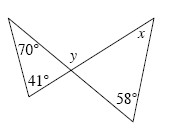2.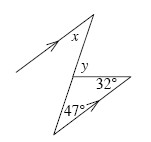3.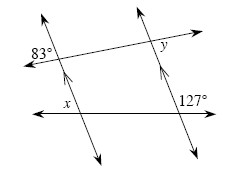4.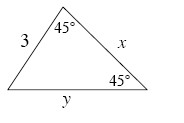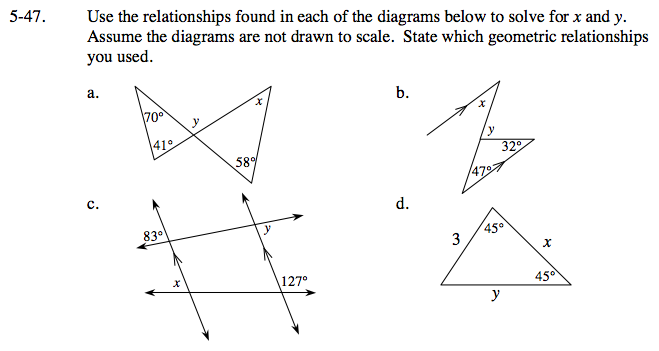Solve for the missing angle in the triangle with 70° and 41°. Use the triangle sum theorem to solve for the missing angle.

41° + 70° + missing angle = 180°

missing angle = 69°

y and the 69° angle are supplementary angles, because they are a linear pair

69° + y = 180°

y = 111°

The 69° angle and the angle across from it are vertical and vertical angles are congruent.

Using the triangle sum theorem and the triangle on the right,
69° + 58° + x = 180° so
x = 122°

y = 79°, x = 47°

Find the measures of any angles that may relate to the unknown angles by using the angles given.

This is a special right triangle. Try drawing it to scale and then solve for x and y.